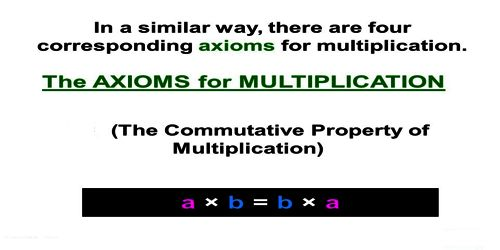Mathematic

# Multiplicative AxiomMultiplicative Axiom: If a = b and c = d then ac = bd. Since multiplication is just repeated addition, the multiplicative axiom follows from the additive axiom.

Multiplication If a = b, then ac = bc. (in equity)

If a < b and c > 0, then ac < bc. (in non-equity)

If a < b and c < 0, then ac > bc.

If a > b and c > 0, then ac > bc.

If a > b and c < 0, then ac < bc

Principles of Multiplication

Multiplication is defined this way:

a × 1 = a

a × b = b × a

(a × b) × c = a × (b × c)

a × (b + c) = (a × b) + (a × c)

This definition consists of four axioms, called the identity, commutative, and associative properties of multiplication, and the distributive property of multiplication over addition.

There are three more properties that are very important for multiplication.  They are:

0 × a = 0,

a × (-1) = -a, and

a × (b – c) = (a × b) – (a × c)

Associative Axiom for Multiplication: In a multiplication expression it does not matter how the factors are grouped.

For example (x * y) * z = x * (y * z)

To remember the associative axioms, it might be helpful to think about the word associate, which as a verb means to interact with a group (maybe you associate with a certain group of friends!). The parentheses are grouping operators, that is, they form groups of numbers and operations. You can see in the example above, the 3 can associate with either the 2 or the 4, but the value of each side is still a product of 24.

Rearrangement Property of Multiplication: The factors in a multiplication expression may be arranged and grouped in any order. This is a combination of the associative and commutative axioms.

For example xyz = x(yz) = z(yx) = y(zx)

Information Source: